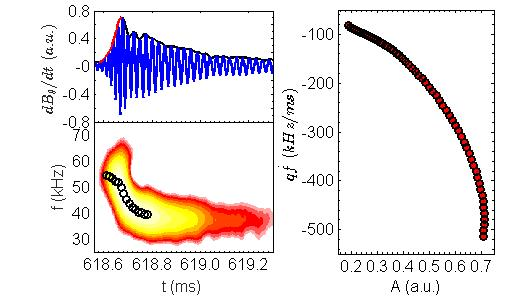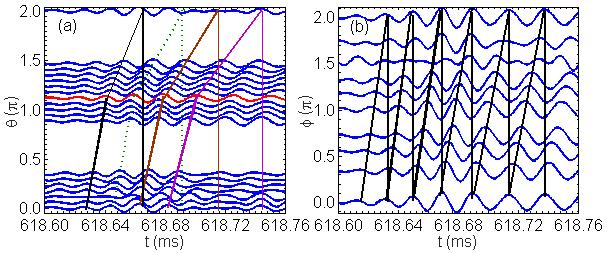Since 18 of December 2019 conferences.iaea.org uses Nucleus credentials. Visit our help pages for information on how to Register and Sign-in using Nucleus.

# 28th IAEA Fusion Energy Conference (FEC 2020)

May 10 – 15, 2021
Virtual Event
Europe/Vienna timezone
The Conference will be held virtually from 10-15 May 2021

## Experimental Evidence of Nonlinear Avalanche Dynamics of Energetic Particle Modes

May 12, 2021, 8:30 AM
4h
Virtual Event

#### Virtual Event

Regular Poster Magnetic Fusion Experiments

### Speaker

Dr Liming Yu (Southwestern Institute of Physics)

### Description

The nonlinear interactions between energetic particle (EP) and Alfvén waves are very important for astrophysics and high temperature plasma physics, especially for magnetically confined fusion plasma, because they will affect the redistribution and transport of EPs significantly. When the EPs have sufficiently strong pressure gradient, they can excite a non-normal mode, named energetic particle mode (EPM) [1-4], which emerges as discrete fluctuation out of the shear Alfvén continuum at the frequency for maximal wave-EP power exchange above the threshold condition due to continuum damping. Therefore, the critical level of tolerable EP losses in a fusion device can be more severe in the presence of EPM.
The early nonlinear theories [5-7] have expected the role of the EPs radial displacements on the time evolution of a strongly driven mode as the EPM. If the EPs move outward, they can locally alter the EP gradient and destabilize a new wave that transports them further, where a new wave is destabilized, much like different runners do in a relay race. The model for the nonlinear evolution is named relay runner model (RRM) . The expected self-consistent nonlinear evolution of the EPM and the EPs have not been unambiguously observed in experiments up to now, because the evolution of the modes are very fast. In this paper, we will give a new experiment evidence about the EPM avalanche in the HL-2A tokamak.The strong EPM instabilities, with frequency chirping-down rapidly and in the range of 35-70 kHz, driven by 42 keV beam ions are observed, immediately, after the turning on of tangential injection NBI. The typical chirping-down process of EPM is shown in the spectrogram of Mirnov signal in Fig.1 (a) and (b). It is obvious that the amplitude of the mode ($A$) increases to the maximum rapidly during t=618.62-618.68 ms, while the mode frequency ($f$) drops rapidly from 54 to 45 kHz. Then, A decreases slowly, and the f decreases to 37 kHz during t=618.70-619.50 ms. The peak fluctuation amplitude $\delta B/B$ is about $(4-5)\times10^{-4}$. The ratio of typical growth time of EPM to Alfvén time is $\tau/\tau_{A}\sim 200$, which is in the expected range where convective EP redistributions can take place [7-9].Evolution of mode numbers near the amplitude rising process is confirmed by the phase shift of the filtered poloidal and toroidal Mirnov waves, as shown in Fig.2. It is obviously that the mode propagates in ion diamagnetic drift direction poloidally. The poloidal mode number changes rapidly from m=2 to 3, and then becomes 4 step by step, which are labeled by the black, brown and pink lines in Fig.2 (a), respectively. The change of m can be completed within 0.06 ms (between t=618.64-618.70ms), and the corresponding frequency changes from 54 to 45 kHz during that time interval. The toroidal mode number is confirmed always as n=1 during the whole chirping down process, as shown in Fig.2 (b). It is indicated that the EPMs move from the core (q=m/n=2, where q is the safety factor) to the edge (q=3 and 4) of plasma, gradually. The EPM mode structure and their evolution in poloidal cross section are obtained by tomography of SXR arrays. The m/n=2/1 mode mainly locates on the core of plasma at first. Then, the modes propagate to the edge gradually. At last, the edge (m=3 and m=4) elements become dominant.
According to the RRM model, for dominant circulating particles driven EPM at transit resonance, as in the HL-2A experiments, the relationship between the EMP chirping rate $\dot{\omega}$ and $A$ satisfies the scaling $A \propto q\dot{\omega}$. The relationship has been obtained by experiment data, as shown in Fig.1 (c). It can be seen that the amplitude of Mirnov signal increases rapidly during t=618.60-618.68 ms, which is labeled by the red line in Fig.1 (a). The center frequency of the mode is labeled by the black circles in Fig.1 (b). The q value timing change rate of the frequency ($\dot{f}$) is roughly proportional to mode amplitude, e.g., $A \propto q\dot{f}$, as shown in Fig.1 (c).
For a single n coherent fluctuation, one obtains, from the conservation of the extended phase space Hamiltonian, $\dot{E}/\dot{r}=-m_i\Omega_i \omega r/m$. On the other hand, from wave-particle phase-locking for maximized power exchange, the derivative of the EPM frequency with time ($\dot{\omega}$) can be expressed as $\dot{\omega}=\dot{\omega_{tr}}\approx -\Omega_{i}r\dot{r}/(nR_0^2q^3)$. Therefore, the radial velocity of outwards energetic ions can be expressed as $\dot{r}=-nR_0^2q^3\dot{\omega}/(\Omega_ir)$. Experimentally, the average radial velocity of the waves ($V_p$) can be estimated by the ratio of the distance from q=2 to 4 rational surfaces ($\Delta r$) to the time interval ($\Delta t$). Compare to the above result, we obtained $|\dot{r}/V_p|\approx O(1)$, i.e., the observed mode radial propagation velocity is comparable to that of Eps, as predicted by RRM model.

This work is supported in part by the National Key R$\&$D Program of Cnina under Grant Nos. 2017YFE0301202 and 2018YFE0304102, and by NNSF of China under Grant Nos. 11875024, 11875021 and 11835010.

Reference:
 L. Chen, Phys. Plasmas 1, 1519 (1994).
 F. Zonca et al., Plasma Phys. Control. Fusion 48, 537 (2006).
 L. Chen and F. Zonca, Review of Mordern Physics 88, 015008 (2016).
 W.W. Heidbrink, Phys. Plasma 15, 055501 (2008).
 R.B. White et al., Phys. Plasmas 26, 2958 (1983).
 F. Zonca and L. Chen, 6th IAEA TCM on Energetic Particles in Magnetic Confinement Systems, Oct. 12-14, 1999, JAERI, Naka, JAPAN.
 L. Chen and F. Zonca, Nucl. Fusion 47, S272 (2007).
 F. Zonca, et al., Nucl. Fusion 45, 477 (2005).
 F. Zonca et al., Plasma Phys. Controlled Fusion 57, 014024 (2015).
 L. Chen et al., Nucl. Fusion 28, 389 (1988).

Country or International Organization China Southwestern Institute of Physics

### Primary author

Dr Liming Yu (Southwestern Institute of Physics)

### Co-authors

Prof. Fulvio Zonca (ENEA, Fusion and Nuclear Safety Department, C. R. Frascati) Prof. Liu Chen (Institute for Fusion Theory and Simulation and Department of Physics, Zhejiang University) Prof. Zhiyong Qiu (Institute for Fusion Theory and Simulation and Department of Physics, Zhejiang University) Prof. Xuantong Ding (Southwestern Institute of Physics) Prof. Wei Chen (Southwestern Institute of Physics) Prof. Xiaoquan JI (Southwestern Institute of Physics) Dr Tao Wang (Key Laboratory of Optoelectronic Devices and Systems of Ministry of Education and Guangdong Province, College of Physics and Optoelectronic Engineering, Shenzhen University) Dr Tianbo Wang (Southwestern Institute of Physics) Prof. Zhongbing Shi (Southwestern Institute of Physics) Prof. Baoshan Yuan (Southwestern Institute of Physics) Prof. Yi Liu (Southwestern Institute of Physics) Prof. Min Xu (Southwestern Institute of Physics)